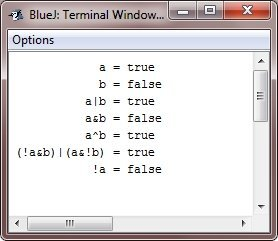# Java Boolean Logical Operators

The Boolean logical operators operates only on boolean operands. All of the binary logical operators combine two boolean values to form a resultant boolean value.

## Boolean Logical Operators List

Here, the table given below, lists the boolean operators available in Java:

Operator Name
& Logical AND
| Logical OR
^ Logical XOR (exclusive OR)
|| Short-circuit OR
&& Short-circuit AND
! Logical unary NOT
&= AND assignment
|= OR assignment
^= XOR assignment
== Equal to
!= Not equal to
?: Ternary if-then-else

The Boolean logical operators, &, |, and ^, operate on boolean value in the same way that they operate on the bits of an integer. The logical unary NOT (!) operator inverts the Boolean state: !true == false and !false == true.

### Java Boolean Logical Operations Effect

Here this table shows the effect of each logical operation:

A B A | B A & B A ^ B !A
False False False False False True
True False True False True False
False True True False True True
True True True True False False

## Boolean Logical Operators Example

Here is an example program of boolean logical operators. Following program almost the same as the BitLogic example shown earlier (in the bitwise operator chapter), but it operate on boolean logical values instead of binary bits:

```/* Java Program Example - Java Boolean Logical Operators
*  Demonstrate the boolean logical operators. */

public class JavaProgram
{
public static void main(String args[])
{

boolean a = true;
boolean b = false;

boolean c = a | b;
boolean d = a & b;
boolean e = a ^ b;
boolean f = (!a & b) | (a & !b);
boolean g = !a;

System.out.println("            a = " + a);
System.out.println("            b = " + b);
System.out.println("          a|b = " + c);
System.out.println("          a&b = " + d);
System.out.println("          a^b = " + e);
System.out.println("(!a&b)|(a&!b) = " + f);
System.out.println("           !a = " + g);

}
}```

When the above Java program is compile and executed, it will produce the following output:### More Examples

Here are some examples listed based on some boolean logical operators, that you can go for. The Equal to (==) operator used most.

Here, don't be confuse with above some examples like reading file, writing to file etc. You will learn all one by one.

Tools
Calculator